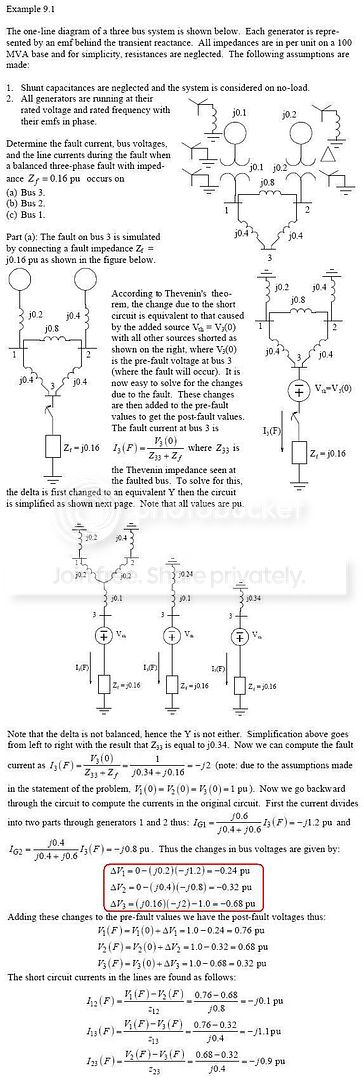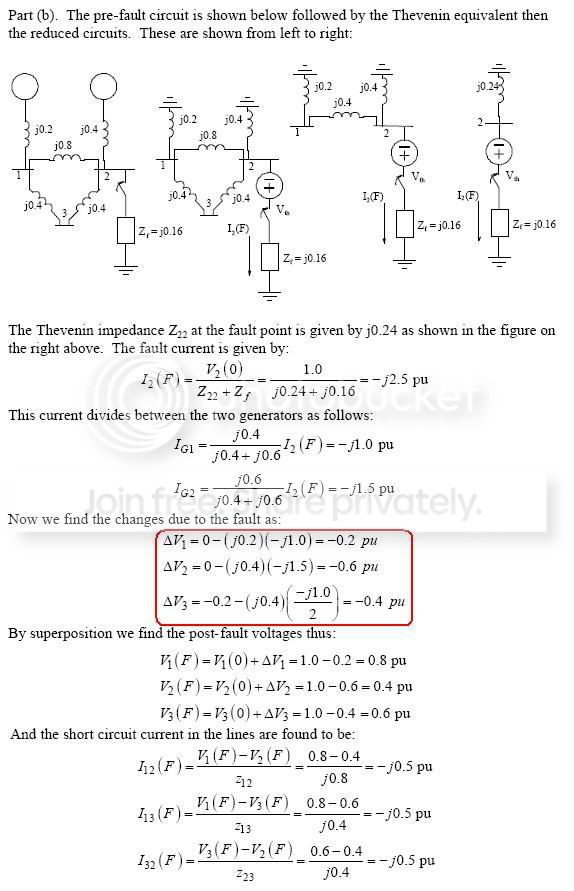# Pls help to explain this 3ph symmetrical fault problem...

Status
Not open for further replies.

#### powersysThe Example 9-1 shown in the attached images was taken from Power System Analysis (2ed, by Hadi Saadat). I do not understand the part marked by the red outline rectangle:

ΔV1 = 0.00 - (j0.20)(-j1.20) = -0.24 pu
ΔV2 = 0.00 - (j0.40)(-j0.80) = -0.32 pu
ΔV3 = (j0.16)(-j2.00) - 1.00 = -0.68 pu

Why "0.00" was used to calculate the ΔV1 and ΔV2? How do the equations above come about? Pls advise.

Thanks.Status
Not open for further replies.